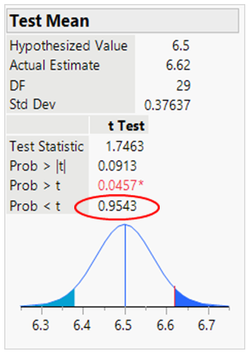Share your ideas for the JMP Scripting Unsession at Discovery Summit by September 17th. We hope to see you there!
Choose Language Hide Translation Bar
[JMP SEA] JMP on Tuesday Session #2 : One mean Tutorial

JMP on Tuesday Session #2, 28th April, 2020

Presenter : Mun Keat Woon ( @MK_Woon )

Topic : One mean Tutorial

Thank you for your questions during the session. We're sharing the answers here for everyone and for us to improve our JMP skills together.

Recorded Video of the session:

Q1 : How to combine multiple actions and broadcasting them to the output reports ?

A1 : The answer is hold down Ctrl and Alt at the same time, click on the red triangle, select the options you want and click OK to generate the reports.

Q2 : Testing the alternative of mean < 6.5 below:

Q: ¬Is it in candy example , mean < 6.5 is higher probability ??¬

Q: ¬So, hypothesis that mean < 6.5 is cannot be rejected .., ¬The answer is we do not reject the null hypothesis since the p-value corresponding to the alternative hypothesis that the mean is < 6.5 is 0.9543 which is larger than the threshold of 0.05. There is insufficient evidence to conclude that the mean filling of candy per bag is less than 6.5 ounces.Q3. When do we use one-sample z statistic?

A3. The one-sample z statistic is used instead of the one-sample t statistic when σ is known.

Q4. The larger or smaller the P-value, the stronger the evidence against the null hypothesis provided by the data?

A4. Smaller.

Q5. What the assumptions are made when you use one sample t test?

A5. 1) The data are continuous (not discrete).

2) The data follow the normal probability distribution.

3) The sample is a simple random sample from its population. Each individual in the population has an equal probability of being selected in the sample.

Article Labels

There are no labels assigned to this post.

Article Tags